# Cox-Ingersoll-Ross Stochastic Clocks

In this post, we consider stochastic clocks whose instantaneous rate of activity follows a square root process. We present all intermediate steps in the derivation of the characteristic function of the corresponding time change. The corresponding steps closely resemble those in the derivation of the zero-coupon bond price in the Cox et al. (1985) model for the short-term interest rate.

In a previous post we introduced the general concept of stochastic clocks. Here, we consider the case where the instantaneous rate of activity process has the dynamics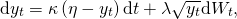where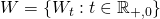is a standard one-dimensional Brownian motion andare constants. The corresponding time-change is given by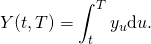Laplace Transform of the Time-Change

We fix a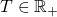and start by computing the Laplace transformWhen, we recognize this as the timeprice of a zero-coupon bond with maturity inin the Cox et al. (1985) model; see e.g. Musiela and Rutkowski (2005). Since solutions to stochastic differential equations have the Markov property, it follows that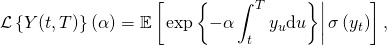whereis the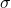-algebra generated by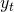. Now, since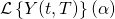is adapted to the filtration, it follows that there exists a function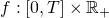such that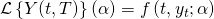.

Note that for, we have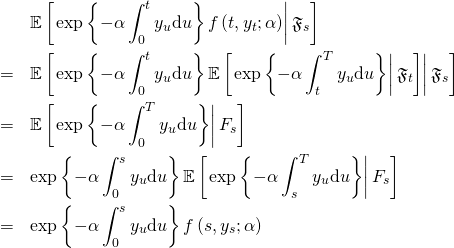It follows that the processdefined asis a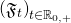-martingale. Using the Itō product rule, we find that its differential is given byHere, we used that the first factor of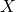is a process of bounded variation. Furthermore, we assume that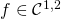so that the Itō formula can be applied. It follows from the martingale property that the drift term in the above differential has to vanish. We obtain the PDEwith terminal condition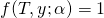for all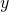. We postulate a solution of the form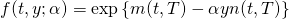whereWe also require thatsuch that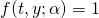for alland the terminal condition is satisfied. Substituting into the PDE yieldsHere, the second step follows frombeing strictly positive. Since this PDE has to hold for all values of, the term that multiplies it has to be equal to zero and we obtain the first ODE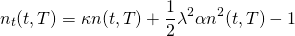with terminal condition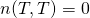. Setting this term equal to zero in the original PDE yields the second ODE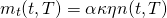with terminal condition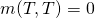.

Solving the ODEs

The first ODE is of the Riccati type and can thus be simplified using the standard transformations for this class. We start by defining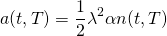such that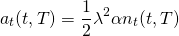and getWe now set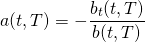such thatand get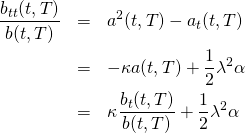orThis is a homogeneous second order linear ODE with constant coefficients and can be solved using standard methods. We note thathas been fixed and make another substitution by definingsuch that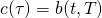withand. We getThe characteristic equation iswith rootsWe thus have the general solution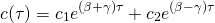with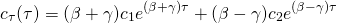and for some constants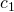and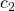to be determined. We obtian the solution to the Riccati ODE by back substitutingBy the terminal condition, it follows thatThus,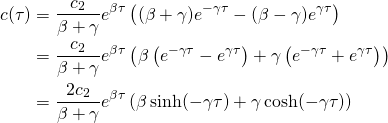and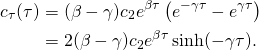We get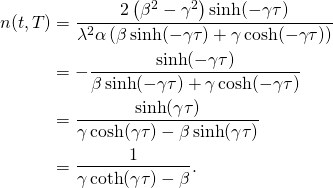To solve for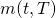, we first note that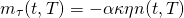which can be solved by integration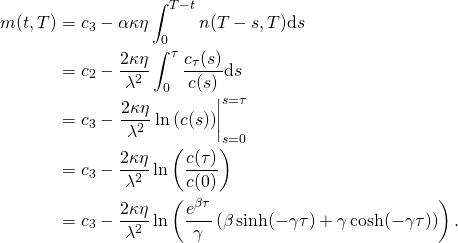By the terminal condition, it follows that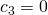and we getPutting Everything Together

Putting everything together, we find that the Laplace transform of the integrated time-change is given byThe characteristic function ofis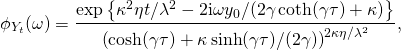where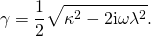This expression is equivalent to the ones given in Carr et al. (2003) and Schoutens (2003), who both define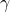slightly differently.

References

Carr, Peter, Hélyette Geman, Dilip B. Madan, and Marc Yor (2003) “Stochastic Volatility for Lévy Processes”, Mathematical Finance, Vol. 13, No. 3, pp. 345-382

Cox, John C., Jonathan Ingersoll Jr., and Stephen A. Ross (1985) “A Theory of the Term Structure of Interest Rates”, Econometrica, VOl. 53, No. 2, pp. 385-407

Musiela, Marek and Marek Rutkowski (2005) Martingale Methods in Financial Modelling: Springer

Schoutens, Wim (2003) Lévy Processes in Finance: John Wiley & Sons